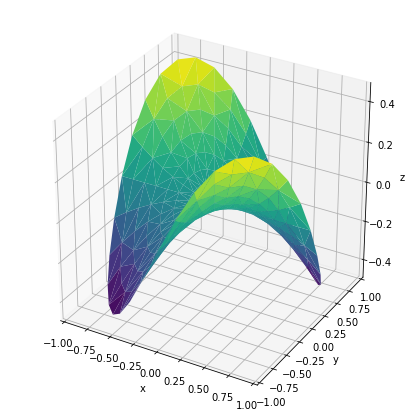# Trisurf3d demo¶

Most examples work across multiple plotting backends, this example is also available for:

## HoloViews¶

In :
import numpy as np
import holoviews as hv
hv.extension('matplotlib')### Define data¶

In :
n_radii = 8
n_angles = 36

# Make radii and angles spaces (radius r=0 omitted to eliminate duplication).
angles = np.linspace(0, 2*np.pi, n_angles, endpoint=False)

# Repeat all angles for each radius.
angles = np.repeat(angles[..., np.newaxis], n_radii, axis=1)

# Convert polar (radii, angles) coords to cartesian (x, y) coords.
# (0, 0) is manually added at this stage,  so there will be no duplicate
# points in the (x, y) plane.

# Compute z to make the pringle surface.
z = np.sin(-x*y)

trisurface = hv.TriSurface((x, y, z))


## Plot¶

In :
trisurface.opts(fig_size=200)

Out: